# Physics: CBSE Sample Question Paper (2020-21)- 1 Notes | Study Physics Class 12 - NEET

## NEET: Physics: CBSE Sample Question Paper (2020-21)- 1 Notes | Study Physics Class 12 - NEET

The document Physics: CBSE Sample Question Paper (2020-21)- 1 Notes | Study Physics Class 12 - NEET is a part of the NEET Course Physics Class 12.
All you need of NEET at this link: NEET

Class-XII
Physics Theory
TIME: 3 Hrs.
M.M: 70

General Instructions:

(1) All questions are compulsory. There are 33 questions in all.
(2) This question paper has five sections: Section A, Section B, Section C, Section D and Section E.
(3) Section A contains ten very short answer questions and four assertion reasoning MCQs of 1 mark each, Section B has two case-based questions of 4 marks each, Section C contains nine short answer questions of 2 marks each, Section D contains five short answer questions of 3 marks each and Section E contains three long answer questions of 5 marks each.
(4) There is no overall choice. However, an internal choice is provided. You have to attempt only one of the choices in such questions.

Section A

All questions are compulsory. In the case of internal choices, attempt any one of them.
Q.1. The figure shows electric field lines in which an electric dipole P is placed as shown. In which direction, the dipole will experience a force? (1 Mark)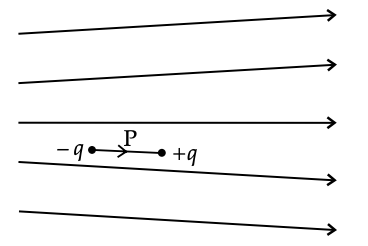Ans: We know the electric field emerges radially outward from the positive point charge. In the figure given above, space between field lines increases (or the density of the electric field line is decreasing). In other words, the electric force is decreasing while moving from left to right. Thus, the force on charge – q is greater than the force on charge + q in turn, a dipole will experience a force towards the left direction.

Q.2. A capacitor of 4 μF is connected, as shown in the figure. The internal resistance of the battery is 0.5 Ω.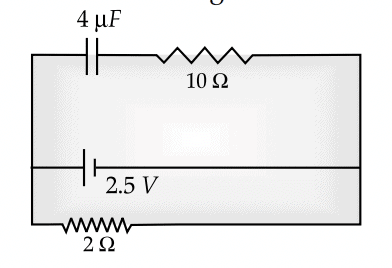Find the amount of charge on the capacitor plates. (1 Mark)
Ans: As the capacitor offers infinite resistance for the DC circuit. The cells current will not flow across the branch of 4 mF, and 10 W. So current will flow across 2-ohm branches. So current flows through across 2 W resistance from left to right is,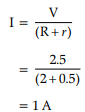So Potential Difference (PD) across 2 W resistance V = RI = 2 × 1 = 2 Volt As battery,
capacitor and 2 branches are in parallel. So PD will remain the same across all three
branches. As current does not flow through the capacitor branch, so no potential drop will be across 10 Ω. So PD across 4 μF capacitor = 2 Volt
Q = CV = 4 μF× 2 V = 8 μC.

OR

What is the value of the phase difference between two points on the same wavefront?   (1 Mark)
Ans: Zero
Detailed answer: The phase difference between two points on a wavefront is zero. The phase difference is defined as the difference in the phase angle between the two waves.

Q.3. The output of a step-down transformer is measured to be 24 V when connected to a 12 W Light bulb. Find the value of the peak current. (1 Mark)
Ans: Given, Power associated with secondary, Ps = 12 W Secondary voltage, Vs = 24 V Current in the secondary, I=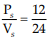= 0.5 A Peak value of the current in the secondary,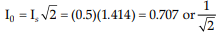A.

Q.4. Two charged particles traverse identical helical paths in an opposite sense in a uniform magnetic field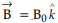. What will be their charge to the mass ratio? (1 Mark)
Ans: When the charge/mass ratio of these two particles are the same and charges on them are of opposite nature, then the charged particles will traverse identical helical paths in a opposite sense.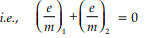OR

Name the phenomenon which shows the quantum nature of electromagnetic radiation.   (1 Mark)
Ans: Photoelectric effect and the Compton effect.

Q.5. If the number of turns per unit length of a coil of a solenoid is doubled, how will its self-induction change?  (1 Mark)
Ans: The self-inductance of coil or solenoid is given by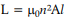where μ0 is the permeability of free space, n is the number of turns per unit length of a coil, A is the cross-sectional area of the coil, and l is the length of the coil.

Q.6. What do you mean by the sensitivity of the meter bridge?  (1 Mark)
Ans: The meter bridge is more sensitive when all the resistances are in the same order or their ratio is unity.

Q.7. A ray of light incident at an angle q on a refracting face of a prism emerges
from the other face normally. If the angle of the prism is 5° and the prism is made of
a material of refractive index 1.5, what is the angle of incidence?  (1 Mark)
Ans: Given that,
A = 5°

μ = 1.5

i= 0°

r2 = 0°
As we know,
Since,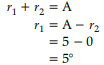From Snell’s law :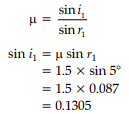∴ i1 angle of incidence = 7.5°.

OR

What is the maximum number of spectral lines emitted by a hydrogen atom in the third excited state?  (1 Mark)
Ans: If n is the quantum number of the highest energy level, then the total number of possible spectral lines emitted is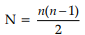Here, third excited state means fourth energy level, i.e., n = 4 ∴ N = 4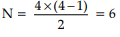Q.8. A cylindrical bar magnet is rotated about its axis in the figure. A wire is connected from the axis and is made to touch the cylindrical surface through contact. Then, find the amount of current flowing through the ammeter. (1 Mark)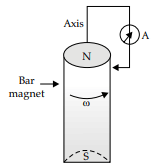Ans: The phenomenon of electromagnetic induction is used in this problem. Whenever the number of magnetic lines of force (magnetic flux) passing through a circuit changes (or a moving conductor cuts the magnetic flux), an emf is produced in the circuit (or emf induces across the ends of the conductor), is called induced emf. The induced emf persists only as long as there is a change or cutting of flux. When a cylindrical bar magnet is rotated about its axis, no change in flux is linked with the circuit. Consequently, no emf induces, and hence, no current flows through the ammeter A. Hence the ammeter shows no deflection.

OR

Find the ratio of de-Broglie wavelengths associated with two electrons accelerated through 25 V and 36 V.  (1 Mark)
Ans: V1 = 25 V, V2 = 36 V
the de-Broglie wavelength of an electron :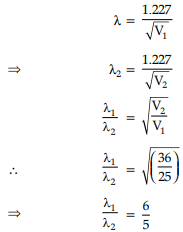Q.9. Name the phenomenon which shows the quantum nature of electromagnetic
Ans: Photoelectric Effect (Raman Effect/Compton Effect)

Q.10. Name the minority charge carriers in n-type silicon.  (1 Mark)
Ans: Holes are the minority carrier in n-type Silicon.

For question numbers 11, 12, 13 and 14, two statements are given-one labelled
Assertion (A) and the other labelled Reason (R). Select the correct answer to these
questions from the codes (a), (b), (c), and (d) as given below:
Q.11. Assertion (A): It is impossible to use 35Cl for fusion.
Reason (R): Binding energy of 35Cl is minimal.   (1 mark)
(a) Both A and R are true, and R is the correct explanation of A
(b) Both A and R are true, but R is NOT the correct explanation of A
(c) A is true, but R is false
(d) A is false, and R is also false
Ans: Correct option is (c)
Explanation: Only lighter atoms are used for fusion. So, 35Cl cannot be used for Fusion. So the assertion is true.
The binding energy of Chlorine binding energy is large. So the reason is false.

Q.12. Assertion(A): If an electron is not deflected when moving through a certain region of space, the only possibility is that no magnetic field is present.
Reason (R): Force on the electron is directly proportional to the strength of the magnetic field  (1 mark)
(a) Both A and R are true, and R is the correct explanation of A
(b) Both A and R are true, but R is NOT the correct explanation of A
(c) A is true, but R is false
(d) A is false, and R is also false
Ans: Correct option is (a)
Explanation: In the absence of a magnetic field, moving electron will not be deflected. This possibility is true.
So, the assertion is true.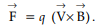So, the electron's force is directly proportional to the magnetic field's strength. So, the reason is true. Reason properly explain the assertion.

Q.13. Assertion (A): When the magnetic flux changes around a metallic conductor, the eddy current is produced.
Reason (R): Electric potential determines the flow of charge.  (1 mark)
(a) Both A and R are true, and R is the correct explanation of A
(b) Both A and R are true, but R is NOT the correct explanation of A
(c) A is true, but R is false
(d) A is false, and R is also false
Ans: Correct option is (b)
Explanation: Change in flux induces emf in the conductor, which generates eddy current. So the assertion is true.
Electric potential determines the flow of charge. So, the reason is also true.
But the reason is not the proper explanation of the generation of eddy current.

Q.14. Assertion (A): Magnetic poles cannot be separated by breaking a bar magnet into two pieces.
Reason (R): When a magnet is broken into two pieces, the magnetic moment will be reduced to half.  (1 mark)
(a) Both A and R are true, and R is the correct explanation of A
(b) Both A and R are true, but R is NOT the correct explanation of A
(c) A is true, but R is false
(d) A is false, and R is also false
Ans: Correct option is (b)
Explanation: Magnetic poles always exist in pairs, even at the atomic level. So the assertion is true.
When a magnet is broken into two pieces, the pole strength remains the same; only the length becomes half. So, the magnetic moment becomes half. So, the reason is also true.
But R is not the proper explanation of A.

Section B

Questions 15 and 16 are Case Study based questions and are compulsory. Attempt any 4 subparts from each question. Each question carries 1 mark.
Q.15. Faster and smaller: The future of computer technology The Integrated Chip (IC) is at the heart of all computer systems. In fact, ICs are found in almost all electrical devices like cars, televisions, CD players, cell phones etc. The miniaturisation that made the modern personal computer possible could never have happened without the IC. IC's are electronic devices that contain many transistors, resistors, capacitors, connecting wires – all in one package. You must have heard of the microprocessor. The microprocessor is an IC that processes all information in a computer, like keeping track of what keys are pressed, running programmes, games etc. The IC was first invented by Jack Kilby at Texas Instruments in 1958, and he was awarded Nobel Prize for this in 2000. IC's are produced on a piece of semiconductor crystal (or chip) by a photolithography process. Thus, the entire Information Technology (IT) industry hinges on semiconductors. Over the years, the complexity of ICs has increased while the size of its features continued to shrink. In the past five decades, dramatic miniaturisation in computer technology has made modern-day computers faster and smaller. In the 1970s, Gordon Moore, co-founder of INTEL, pointed out that the memory capacity of a chip (IC) approximately doubled every one and a half years. This is popularly known as Moore’s law. The number of transistors per chip has risen exponentially, and each year, computers are becoming more powerful yet cheaper than the year before. It is intimated from current trends that the computers available in 2020 will operate at 40 GHz (40,000 MHz) and would be much smaller, more efficient and less expensive than present-day computers. A famous quote from Gordon Moore best expresses the explosive growth in the semiconductor industry and computer technology: “If the auto industry advanced as rapidly as the semiconductor industry, a Rolls Royce would get half a million miles per gallon, and it would be cheaper to throw it away than to park it”.

1. Full form of IC is: (1 mark)
(a) Indigenous circuit
(b) Improved chip
(c) Isolated circuit
(d) Integrated chip
Ans: Correct option is (d)
Explanation: The full form of IC is an integrated chip or Integrated Circuit.
2. IC was first invented by (1 mark)
(a) Isaac Newton
(b) W. H. Schottky
(c) Charles Babbage
(d) Jack Kilky
Ans: Correct option is (d)
Explanation: The IC was first invented by Jack Kilby at Texas Instruments in 1958, and he was awarded Nobel Prize for this in 2000.
3. Moor’s Law states that: (1 mark)
(a)
The memory capacity of a chip (IC) approximately doubles every one and a half years
(b)
The packing density doubles every year.
(c)
The memory capacity of a chip (IC) approximately doubles every two and a half years
(d)
Operational frequency of computer doubles every one and a half years.
Ans: Correct option is (a)
Explanation: In the past five decades, dramatic miniaturisation in computer technology has made modern-day computers faster and smaller. In the 1970s, Gordon Moore, co-founder of INTEL, pointed out that a chip (IC) memory capacity approximately doubled every one and a half years. This is popularly known as Moore’s law.
4. Which statement is correct?  (1 mark)
(a) IC's contain many transistors, resistors, capacitors, connecting wires.
(b) IC's contain many transistors, resistors, capacitors, inductors, connecting wires
(c) IC's contain many transistors, capacitors, inductors, connecting wires.
(d) IC's contain many transistors, resistors, capacitors, crystal oscillator, connecting wires.
Ans: Correct option is (a)
Explanation: IC's are electronic devices that contain many transistors, resistors, capacitors, connecting wires – all in one package. It does not contain any inductor or crystal oscillator.
5. IC's are produced on a piece of semiconductor crystal by a process called (1 mark)
(a)
X-ray imaging
(b)
Ultrasonography
(c)
Magnetic resonance imaging
(d)
photolithography
Ans: Correct option is (d)
Explanation: IC's are produced on a piece of semiconductor crystal (or chip) by a photolithography process.
Q.16. Photocell: A photocell is a technological application of the photoelectric effect. It is a device whose electrical properties are affected by light. It is also sometimes called an electric eye. A photocell consists of a semi-cylindrical photo-sensitive metal plate C (emitter) and a wire loop A (collector) supported in an evacuated glass or quartz bulb. It is connected to the external circuit having a high-tension battery B and microammeter (μA) as shown in the Figure.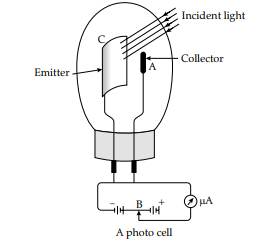Sometimes, instead of plate C, a thin layer of photosensitive material is pasted on the inside of the bulb. A part of the bulb is left clean for the light to enter. When the light of suitable wavelength falls on the emitter C, photoelectrons are emitted. These photoelectrons are drawn to the collector A. Photocurrent of a few microampere order can be normally obtained from a photocell. A photocell converts a change in intensity of illumination into a photocurrent change. This current can be used to operate control systems and light measuring devices.

1. Photocell is also known as  (1 mark)
(a) Electric sense
(b) Electric eye
(c) Photo emitter
(d) Photo transducer
Ans: Correct option is (b)
Explanation: A photocell is a technological application of the photoelectric effect. It is a device whose electrical properties are affected by light. It is also sometimes called an electric eye.

2. A photocell consists of (1 mark)
(a) a semi-cylindrical photo-sensitive metal plate called emitter and a wire loop called collector
(b) A metal cylinder called emitter and a filament called the collector.
(c) Two semi-cylindrical photo-sensitive metal plates – one is called emitter, and the other is called collector
(d) A wire mesh called emitter and a photosensitive wire loop called collector
Ans: Correct option is (a)
Explanation: A photocell consists of a semi-cylindrical photosensitive metal plate C (emitter) and a wire loop A (collector) supported in an evacuated glass or quartz bulb.

3. Which of the following statements is true?  (1 mark)
(a) The photocell is totally painted black
(b) A part of the photocell is left clean
(c) The photocell is completely transparent.
(d) A part of the photocell is made black
Ans: Correct option is (b)
Explanation: A part of the bulb is left clean for the light to enter.

4. The photocurrent generated is in the order of  (1 mark)
(a) Ampere
(b) Milliampere
(c) Microampere
(d) None of the above
Ans: Correct option is (c)
Explanation: Photocurrent of the order of a few microamperes can be normally obtained from a photocell.

5. A photocell converts a change in ________ of incident light into a change in ___________.  (1 mark)
(a) Intensity, photo-voltage
(b) Wavelength, photo-voltage
(c) Frequency, photo-current
(d) Intensity, photo-current
Ans: Correct option is (d)
Explanation: A photocell converts a change in intensity of illumination into a photocurrent change.

Section C

All questions are compulsory. In the case of internal choices, attempt any one.
Q.17. State the underlying principle of a transformer. How is the large scale
transmission of electric energy over long distances done using
transformers?   (2 Mark)
Ans: A transformer is based on the principle of mutual induction, which states that due to
continuous change in the current in the primary coil, an emf gets induced across the
secondary coil.
Electric power generated at the power station is stepped-up to very high voltages using a step-up transformer and transmitted to a distant place. At receiving end, it is
stepped down by a step-down transformer.

Q.18. Given a uniform electric field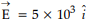N/C. Find the flux of this field through a square of a side 10 cm on a side whose plane is parallel to the y-z plane. What would be the flux through the same square if the plane makes a 30° angle with the x-axis?   (2 Mark)
Ans: Given :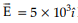N/C along (+) positive direction of x-axis.

Surface area, A = 10 cm × 10 cm

= 0.10 m × 0.10 m

= 10–2 m2
(i) In case of plane parallel to y-z plane, normal to plane will be along x-axis, so ϕ = 0°
Electric flux will be calculated using ϕ =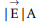cos θ
5 × 103 × 10–2 × cos 0°
= 50 Nm2 /C
(ii) Since the plane is making an angle of 30° with the x-axis, so normal to its plane will make 60° with the x-axis, so θ = 60°
Now finding Electric flux again with ϕ =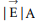cos θ
= 5 × 103 × 10–2 × cos 60°
= 25 Nm2 /C

OR

Why do we prefer carbon brushes to copper in an ac generator?   (2 Mark)
Ans: The carbon brushes used in the generator are corrosion-free. On small
expansion on heating, it maintains the proper contact as well.

Q.19. If magnetic monopoles existed, how would the Gauss law of magnetism be modified?   (2 Mark)
Ans: Gauss law of magnetism describes that divergence of the magnetic field will be zero while divergence of the electric field is not zero, which shows the non-existence of magnetic monopole. As per Gauss law of magnetism,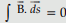If monopole exists, then the right side will be equal to the monopole multiplied by μ0.
Detailed Answer: According to the Gauss law of magnetism,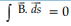(Integral form)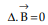(Differential form)
If magnetic monopoles exist, then Gauss's law for magnetism would be modified as :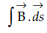(Integral form)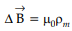(Differential form)
Where, ρm = magnetic charge density μ0 = permeability of free space.

OR

Calculate the amount of work done to dissociate a system of three charges 1 μC, 1 μC and – 4 μC placed   (2 Mark)
Ans: Given :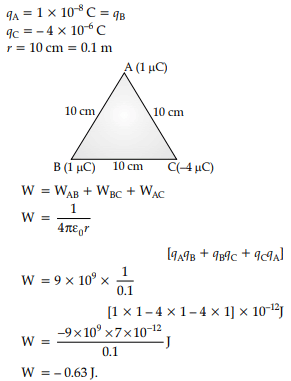Q.20. The battery remains connected to a parallel plate capacitor, and a dielectric slab is inserted between the plates. What will be the effect on its
(i) potential difference
(ii) capacity
(iii) electric field and
(iv) energy stored   (2 Mark)
Ans: When a battery remains connected,
(i) the potential difference V remains constant
(ii) capacity C increases
(iii)the electric field will remain the same
(iv) energy stored 1/2 CV2 increases as C increases
Detailed Answer: When a battery remains connected to a parallel plate capacitor and if a dielectric slab is inserted between the capacitor plates, then
(i) there will be no change in the potential difference as the capacitor remained connected with the battery.
(ii) capacity or capacitance will increase since with the introduction of the dielectric slab, the capacitor's capacitance will result in C =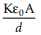where K > 1, increasing C.
(iii)Electric field will remain the same as there will be no change in potential difference and distance between the plates.
(iv) Energy stored will be increased since from the expression U = 1/2 CV2, potential
difference V remains the same while C increases, which finally increases the capacitor's energy.

Q.21. The diagram below shows a potentiometer set up. The galvanometer pointer deflects to the left on touching the jockey near the end X of the potentiometer wire. On touching the jockey near to end Y of the potentiometer, the galvanometer pointer again deflects to the left but now by a larger amount. Identify the fault in the circuit and explain how it leads to such a one-sided deflection using appropriate equations or otherwise.   (2 Mark)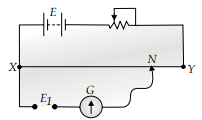Ans: The positive of E1 is not connected to terminal X.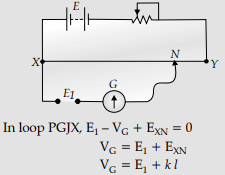So, VG (or deflection) will be maximum when l is maximum, i.e., when a jockey is touched
near and Y. Also, VG (or deflection) will be minimum when l is minimum, i.e., when a jockey is touched near end X.

Q.22. Define the distance of the closest approach. An α-particle of kinetic energy ‘K’ is bombarded on a thin gold foil. The distance of the closest approach is ‘r’. What will be the distance of the closest approach for an α-particle of double the kinetic energy?   (2 Mark)
Ans: It is the distance of the charged particle from the centre of the nucleus, at which the whole of the initial kinetic energy of the (far off) charged particle gets converted into the electric potential energy of the system. 1 Distance of closest approach (rc) is given by rc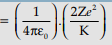'K' is doubled, ∴ rbecomes r/2.
[Alternatively: If a candidate writes directly r/2 without mentioning formula, award the 1 mark for this part.]
Detailed Answer: When an α-particle is bombarded towards the nucleus, it is repelled by electrostatic repulsion. As a result, its kinetic energy is converted into electrostatic potential energy. At a certain distance (rC) between the ∝particle and nucleus, the moving particle loses all its kinetic energy and becomes stationary momentarily. This distance is known as the distance of the closest approach. In this process, the particle's total kinetic energy is converted into potential energy.
Kinetic energy = K =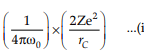Let rC’ be the new distance of closest approach when kinetic energy becomes 2K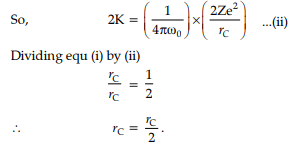Q.23. Write two important limitations of Rutherford's nuclear model of the atom.   (2 Mark)
Ans: (i) According to Rutherford's model, an electron orbiting around the nucleus continuously radiates energy due to the acceleration; hence the atom will not remain stable.
(ii) As electron spirals inwards, its angular velocity and frequency change continuously; therefore, it will emit a continuous spectrum.

Q.24. Calculate the curvature radius of an equi-concave lens of refractive index 1.5, when kept in a medium of refractive index 1.4, to have a power of –5D.  (2 Mark)
Ans: Calculation of focal length
Lens maker's formula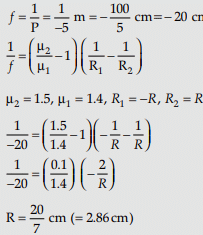Detailed Answer: Given the refractive index of the biconcave lens = 1.5
Power of biconcave lens = – 5D
Refractive index of medium= 1.4.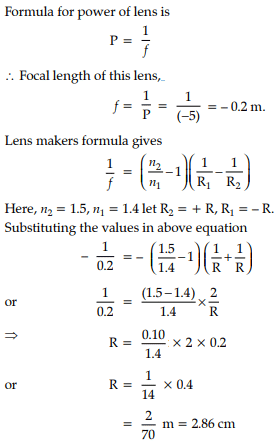OR

A circular coil of cross-sectional area 200 cm2 and 20 turns is rotated about the vertical diameter with an angular speed of 50 rad s–1 in a uniform magnetic field of magnitude 3.0 × 10–2 T. Calculate the maximum value of the current in the coil.   (2 Mark)
Ans: Maximum value of emf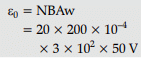= 600 mV
Maximum induced current,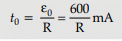Q.25. A rectangular coil of sides ‘l’ and ‘b’ carrying a current I is subjected to a uniform magnetic field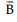, acting perpendicular to its plane. Obtain the expression for the torque acting on it.   (2 Mark)
Ans: Equivalent magnetic moment of the coil,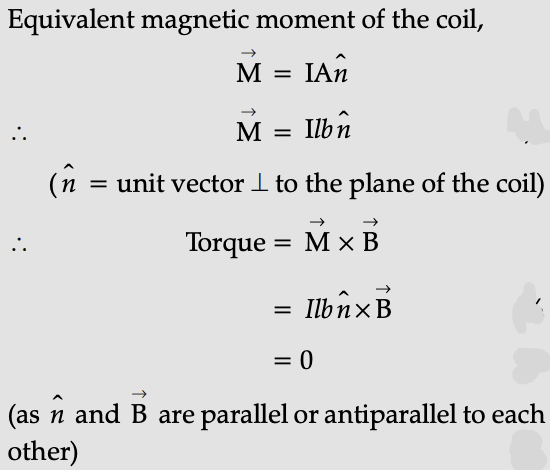Section D

All questions are compulsory. In the case of internal choices, attempt anyone.
Q.26. The figure shows a metallic rod PQ of length l, resting on the smooth horizontal rails AB positioned between a permanent magnet's poles. The rails, the rod, and the magnetic field are in three mutually perpendicular directions. A galvanometer G connects the rails through a switch K. Assume the magnetic field to be uniform. Given the resistance of the closed-loop containing the rod is R.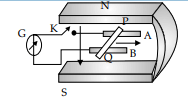(i) Suppose K is open and the rod is moved with a speed v in the direction shown. Find the polarity and magnitude of induced emf.
(ii) With K open and the rod moving uniformly, there is no net force on the rod PQ electrons even though they do experience a magnetic force due to the rod's motion. Explain.
(iii) What is the induced emf in the moving rod if the magnetic field is parallel to the rails instead of perpendicular?   (3 Mark)

Ans:  (i) |ε| = Bvl
P is positive end Q is the negative end
(ii) Magnetic force gets cancelled by electric force that generates an extra charge of opposite sign at rod ends.
(iii)Induced emf is zero as the motion of rod not cutting field lines

Q.27. Define electric flux and write its SI unit. The electric field components in the figure shown are : Ex = αx, Ey = 0, Ez = 0 where α = 100 N/C m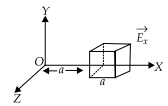Calculate the charge within the cube, assuming a = 0.1 m.   (3 Mark)
Ans: Definition of Electric flux
SI unit
Formula (Gauss's Law)
Calculation of Charge within the cube
Electric Flux is the dot product of the electric field and area vector.
also, accept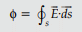SI Unit: Nm2 /C or volt-meter
For a given case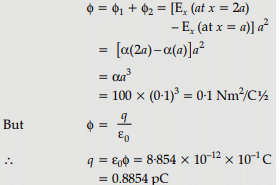OR

(i) Monochromatic light of wavelength 589 nm is incident from the air on a water surface. If μ for water is 1·33, find the refracted light's wavelength, frequency, and speed.
(ii) A double convex lens is made of a glass of refractive index 1·55, with both faces of the same radius of curvature. Find the radius of curvature required if the focal
length is 20 cm.   (3 Mark)
Ans: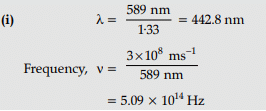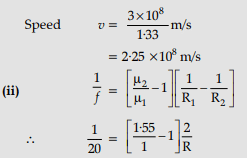Therfore, R = (20 × 1.10) cm = – 22 cm

Q.28. A toroidal solenoid of mean radius 20 cm has 4000 turns of wire wound on a ferromagnetic core of relative permeability 800. Calculate the magnetic field in the core for a current of 3 A passing through the coil. How does the field change when a core of Bismuth replaces this core?   (3 Mark)
Ans: Formula for the magnetic field of a toroid
Calculation of magnetic field
Effect of change of core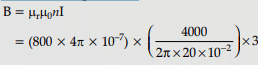= 9.6 T
Since Bismuth is diamagnetic, its μr <
Therefore, the magnetic field in the core will get very much reduced.
Given :
Mean radius of toroidal solenoid = 20 cm
Number of turns of wire wound = 4000
Relative permeability of ferromagnetic core = 800
Current passing through the coil = 3 A
The magnetic field in a toroid coil :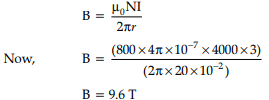As Bismuth is a diamagnetic substance with a relative permeability of less than 1, it will tend to move away from the stronger to the weak part of the external magnetic field, making the core field less than the empty core field.

OR

(i) How are electromagnetic waves produced? Explain.
(ii) A plane electromagnetic wave travels through a medium along the +ve z-direction. Depict the electromagnetic wave showing the oscillating electric and magnetic fields' directions.   (3 Mark)
Ans: (i) Production of EM wave: Electromagnetic waves consist of both electric and magnetic fields travelling through space with the speed of light c. These waves oscillate in perpendicular planes with respect to each other and are in phase. An electromagnetic wave can be created by accelerating charges, moving charges back and forth, producing oscillating electric and magnetic fields. When the accelerating charged particle moves with acceleration, magnetic and electric fields change continuously, leading to the production of electromagnetic waves.
(ii)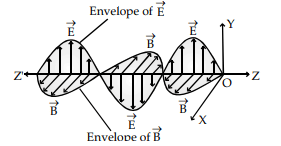Q.29. What is relaxation time? Derive an expression for resistivity of a wire in terms
of the number density of free electrons and relaxation time.   (3 Mark)
Ans: Definition and Derivation.
Detailed answer: (i) Relaxation time shows the effect of collisions among the electrons
and ions or impurities on electrical conduction in a metal. The time is taken for the drift velocity to decay 1/e of its initial value. As drift velocity increases, relaxation time
decreases since the electrons move the distance they frequently collide faster.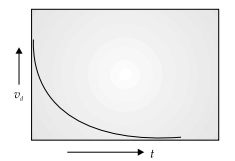(ii) When a potential difference V is applied across a conductor of length l, then drift speed of electron will result as :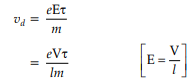The electric current through the conductor and drift speed are linked as I = neAvd
where,
n = number density of electrons
e = electronic charge
A = area of cross-section
vd = electron drift speed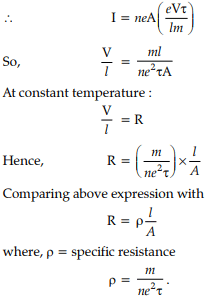Q.30. (i) Three-point charges q, – 4q and 2q are placed at the vertices of a 3 equilateral triangle ABC of side ‘l’ as shown in the figure. Obtain the expression for the magnitude of the resultant electric force acting on the charge q.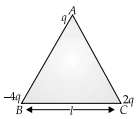(ii) Find out the amount of the work done to separate the charges at infinite distance.   (3 Mark)
Ans: (i) Finding the magnitude of the resultant force on charge q
Force on charge q due to the charge – 4q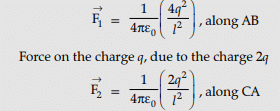The forces F1 and F2 are inclined to each other at an angle of 120°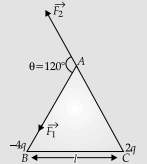Hence, resultant electric force on charge q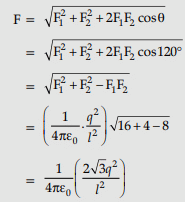(ii) Finding the work done
Net P.E. of the system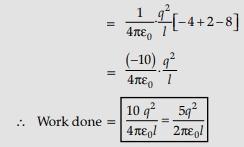Section E

All questions are compulsory. In the case of internal choices, attempt any one.

Q.31. (a) Why are photodiodes preferably operated under reverse bias when the current in the forward bias is known to be more than that in reverse bias?
(b) The two optoelectronic devices: Photodiode and solar cell, have the same working principle but differ in terms of their operation process. Explain the difference between the two devices in terms of :
(i) biasing
(ii) junction area
(iii) I-V characteristics.    (5 Mark)
Ans: (a) The fractional change in majority charge carriers is very less compared to the
the fractional change in minority charge carriers on illumination.
(b) The difference in the working of two devices :

 Photodiode Solar cell (i) Biasing Used in reverse biasing No external biasing is given (ii) Junction Area Small Large for solar radiation to be incident on it.

(iii)I-V characteristics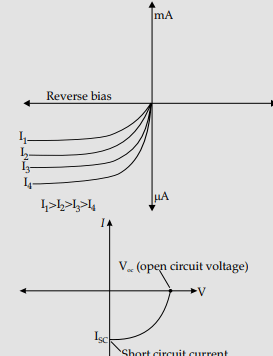OR

(i) Using Bohr’s postulates, derive the expression for the electron's total energy in the stationary states of the hydrogen atom.
(ii) Using Rydberg's formula, calculate the wavelengths of the spectral lines of the first member of the Lyman series and Balmer series.    (5 Mark)
Ans: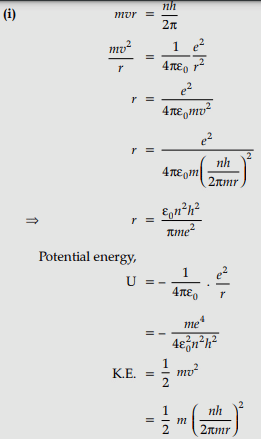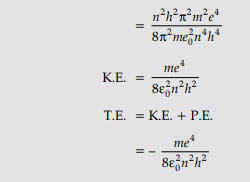(ii) Rydberg's formula: For the first member of the Lyman series,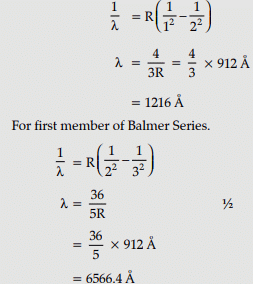Q.32. (i) State Faraday’s laws of electromagnetic induction.
(ii) The magnetic field through a circular loop of wire 12 cm in radius and 8.5 W resistance changes with time, as shown in the figure. The magnetic field is perpendicular to the plane of the loop. Calculate the induced current in the loop and plot it as a function of time.(iii) Show that Lenz’s law is a consequence of conservation of energy   (5 Mark)
Ans: (i) Faraday’s Laws of Electromagnetic Induction:
Faraday’s First Law of Electromagnetic Induction states that whenever a conductor is placed in a varying magnetic field, emf is induced, which is known as induced emf. If the conductor circuit is closed, the current is also induced, called induced current. Faraday’s Second Law of Electromagnetic Induction states that the induced emf is equal to the rate of change of flux linkage where flux linkage is the product of the number of turns in flux associated with the coil.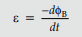eB is the magnetic flux through the circuit as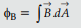With N loops of similar area in a circuit and ϕB being the flux through a loop, then emf is induced in every loop, making Faraday law as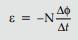where, e = Induced emf [V],
N = number of turns in the coil
∆ϕ= change in the magnetic flux [Wb],
= change in time [s]
The negative sign means that e opposes its cause.
(ii)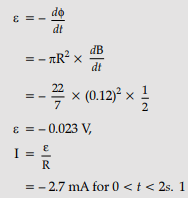(iii) Similarly :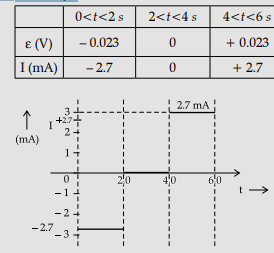Lenz's Law: The polarity of induced emf is such that it tends to produce a current which opposes the change in magnetic flux that produced it.
Explanation :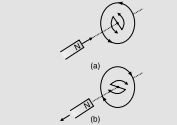When the north pole of a bar magnet is pushed towards the close coil, the magnetic flux through the coil increases and the current is induced in the coil in such a direction that it opposes the increase in flux. This is possible when the coil's induced current is in the anti-clockwise direction. The opposite will happen when the north pole is moved away from the coil. In either case, it is the work done against the force of magnetic repulsion/attraction that gets ‘converted‘ into the induced emf. So Lenz's law is a consequence of the conservation of energy.

OR

(a) Write the expression for the equivalent magnetic moment of a planar current loop of area A, having N turns and carrying a current i. Use the expression to find the magnetic dipole moment of a revolving electron.
(b) A circular loop of radius r, having N turns and carrying current I, is kept in the XY plane. It is then subjected to a uniform magnetic field to Obtain an expression for the magnetic potential energy of the coil-magnetic field system.    (5 Mark)
Ans: (a) The equivalent magnetic moment is given by μ = NiA
The direction of m is perpendicular to the plane of the current-carrying loop. It is directed along the direction of advance of a right-handed screw rotated along the current flow direction.
Derivation of expression for μ of an electron revolving around a nucleus
For 1 revolution of electron, N = 1
Current =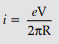Putting in the expression,
μ= NiA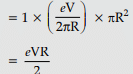(b) for the loop,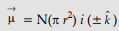Magnetic potential energy =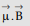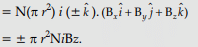Q.33. (i) Derive an expression for drift velocity of electrons in a conductor. Hence deduce Ohm’s law.
(ii) A wire whose cross-sectional area is increasing linearly from one end to another is connected across a V volts battery. Which of the following quantities remain constant in the wire?
(a)
drift speed
(b) current density
(c) electric current
(d) electric field

Ans: (i) Derivation of the expression for drift velocity
Deduction of Ohm's law
Let an electric field E be applied to the conductor. The acceleration of each electron is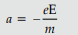Velocity gained by the electron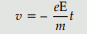Let the conductor contain n electrons per unit volume. The average value of time 't', between their successive collisions, is the relaxation time, 't'.
Hence average drift velocity, vd =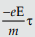The amount of charge, crossing area A, in time Δt, is = neAvdΔt = IΔt
Substituting the value of vd, we get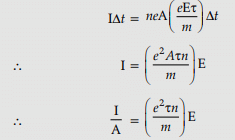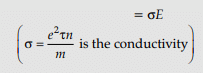But I = JA, where J is the current density,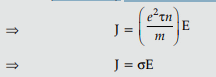This is Ohm's law
[Note: Credit should be given if the student derives the alternative form of Ohm's law by substituting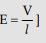(ii) Name of quantity and justification
(b) Current density will remain constant in the wire.
All other quantities depend on the cross-sectional area of the wire.
Detailed Answer: Out of these, current density remains constant in a wire whose cross-sectional area increases linearly from its one end to other as current density is :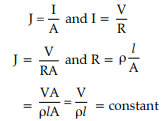It is a current per unit area that depends on the area of cross-section.
Drift speed is given as :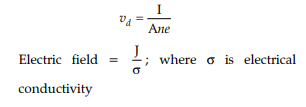OR

A capacitor of capacitance C1 is charged to a potential V1 while another capacitance C2 is charged to a potential difference V2. The capacitors are now disconnected from their respective charging batteries and connected in parallel to each other
(i) Find the total energy stored in the two capacitors before they are connected.
(ii) Find the total energy stored in the parallel combination of the two capacitors.
(iii) Explain the reason for the difference of energy in parallel combination compared to the total energy before they are connected.    (5 Mark)

Ans: (i) Finding the total energy before the capacitors are connected
(ii) Finding the total energy in the parallel combination
(iii)Reason for difference

(i) We have Energy stored in a capacitor = 1/2 CV2
Therefore, Energy stored in the charged capacitors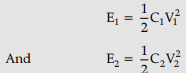Therefore, the total energy stored =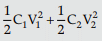(ii) Let V be the potential difference across the parallel combination. Equivalent capacitance = (C+ C2)
Since charge is a conserved quantity, we have (C1 + C2)V = C1V1 + C2V2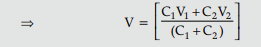Therefore, the total energy stored in the parallel combination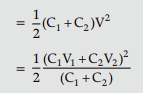(iii) The total energy of the parallel combination is different (less) from the total energy before the capacitors are connected. This is because some energy gets used up due to the movement of charges.

The document Physics: CBSE Sample Question Paper (2020-21)- 1 Notes | Study Physics Class 12 - NEET is a part of the NEET Course Physics Class 12.
All you need of NEET at this link: NEETUse Code STAYHOME200 and get INR 200 additional OFF

## Physics Class 12

134 videos|388 docs|213 tests

### How to Prepare for NEET

Read our guide to prepare for NEET which is created by Toppers & the best Teachers

Track your progress, build streaks, highlight & save important lessons and more!

,

,

,

,

,

,

,

,

,

,

,

,

,

,

,

,

,

,

,

,

,

;